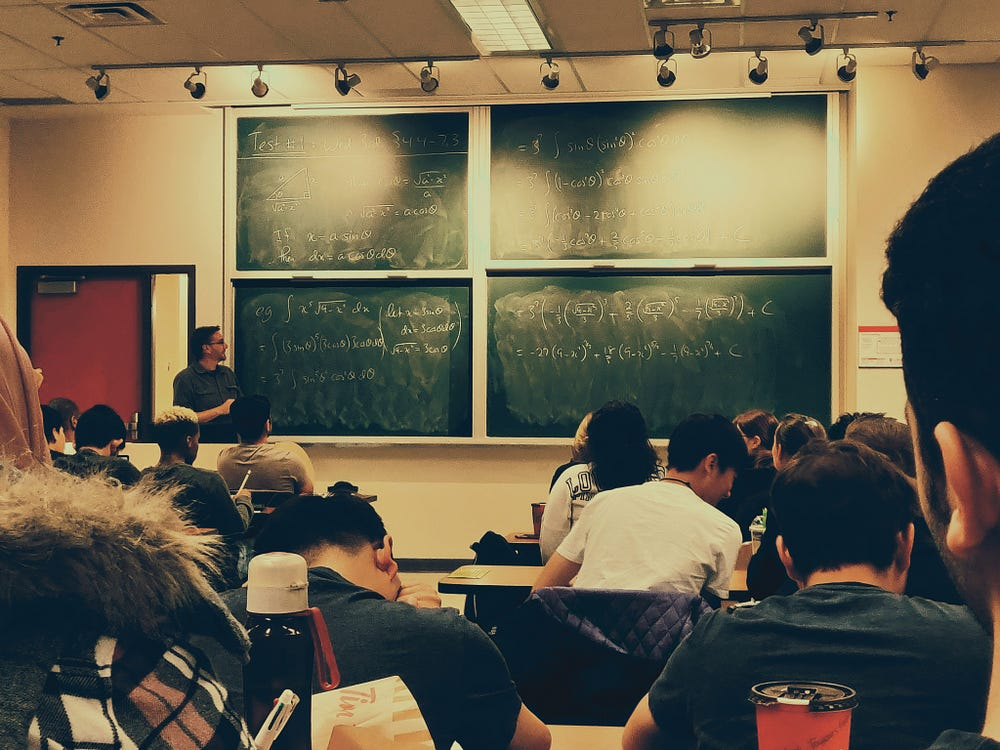# Foundation of Bayesian Learning

Source: Deep Learning on MediumLet us understand Bayes theorem in the context of machine learning. In machine learning we are often looking for the best hypothesis.

Before we proceed any further let us consider a hypothetical example. We have been given a training dataset (say D) which has weight, height and age of a person as attributes and we have to predict whether the person is fit/unfit i.e Boolean classification.

Let H be the set of all possible hypothesis for a given problem. It means that H will have all the permutations and combinations of the attributes(weight, height and age) useful to predict whether a person is fit or unfit. We need to search the best hypothesis in this set H.

From this set of H we will choose a probable hypothesis ‘h’. We will choose this hypothesis based on some initial knowledge of the problem. This initial knowledge that how probable is ‘h’ to be the best hypothesis is known as Prior probability. In case prior knowledge is unavailable, we can assign same probability to all the hypothesis in set H.

We will also need the probability of training data without the knowledge of hypothesis that the data might hold. This probability is P(D).

You might have a question in your mind. Believe me, that is a constraint of Bayesian Learning. That is, you need to have initial knowledge of many probabilities. This is because we are trying to predict Posterior probability using Prior!

P(D|h) will denote the likelihood. In other words, the probability of observing the data according to the given hypothesis ‘h’. P(h|D) is known as Posterior probability. It gives the probability for the hypothesis given the dataset.

We have all the necessary tools to construct Bayes Theorem, a cornerstone of Bayesian Learning —

This is quite intuitive to expect that P(h|D) increases with the high prior knowledge i.e P(h) and with high likelihood P(D|h). Similarly high value of P(D) will reduce P(h|D) because it signals that more data was observed independent of the hypothesis ‘h’.